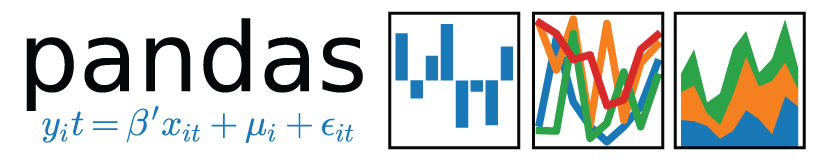# Parallelize Pandas map() and apply() while accounting for future recordsA few blog posts ago, I covered how to parallelize Pandas map() and apply(). You can read more about it at http://blog.adeel.io/2016/11/06/parallelize-pandas-map-or-apply/ … Essentially it works by breaking the data into smaller chunks, and using Python’s multiprocessing capabilities you call map() or apply() on the individual chunks of data, in parallel.

This works great, but what if it’s time series data, and part of the data you need to process each record lies in a future record? For example, if you are tracking the change of price from one moment to what it will be in a moment in the future. In this case the approach I laid out about dividing it into chunks will not work, because as you reach the end of a chunk, you will not have the future records to use.

It turns out that there’s a relatively simple way to do this. Essentially you determine how much in the future you need to go, and include those extra records in each chunk (so some records at the edges are duplicated in chunks), and then drop them at the very end.

So let’s say for each record, you also need records from up to 30 seconds in the future, for your calculation. And each record in your data represents 1 second. So essentially you include 30 extra records in each chunk so they are available for the parallel calculations. And then drop them later.

You start by setting up your parallel processor function like so:

```import pandas as pd
import multiprocessing

cpu_count = multiprocessing.cpu_count()

def parallelize(data, split_interval):
splits = range(0, cpu_count)
parallel_arguments = []
for split in splits:
parallel_arguments.append([split, data, split_interval])
pool = multiprocessing.Pool(cpu_count)
data_array = pool.map(worker, parallel_arguments)
pool.close()
pool.join()
final_data = pd.concat(data_array)
final_data = final_data.groupby(final_data.index).max() #This is where duplicates are dropped.
return final_data.sort_index()
```

What you’ve done is defined an array of a tuple of arguments (parameters) that can are iterated over, to spawn each parallel worker. In the tuple we pass a reference to the Pandas DataFrame, and the data chunk the worker function should work on. Note that the worker function returns that chunk, and concatenates it back into a final DataFrame. After doing is, note the groupby() function that is called, this is where we drop the duplicate records at the edges that were included in each chunk.

Here’s what your worker would do to work on its chunk:

```def worker(params):
num = params
data = params
split_interval = params
split_start = num*split_interval
split_end = ((num+1)*split_interval)+30
this_data = data.iloc[split_start:split_end].copy()
# ...do work on this_data chunk, which includes records from 30 seconds in the future
# Add new columns to this_data, or whatever
return this_data
```

Note this line: split_end = ((num+1)*split_interval)+30. In the chunk you’re working on, you’re including the next 30 records, which in this example represent the next 30 seconds that you need in your calculations.

And finally to tie it together, you do:

```if __name__ == '__main__':